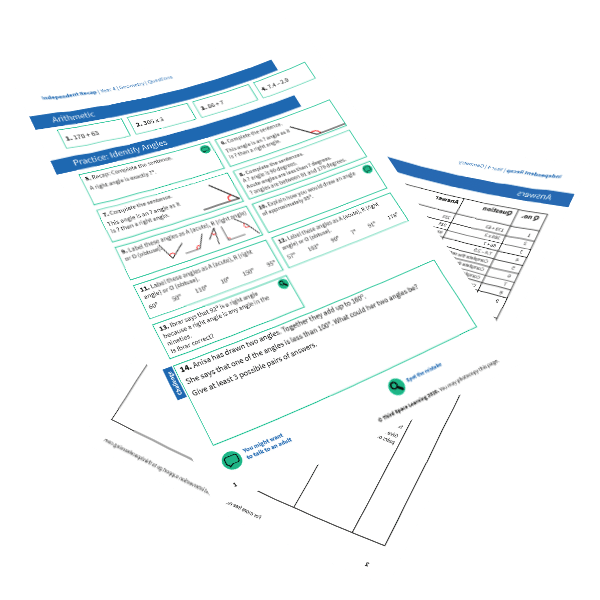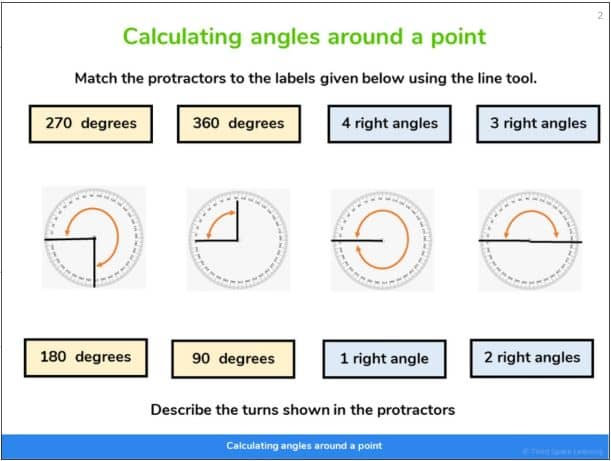Teaching support from the UK’s largest provider of in-school maths tuitionone to one lessonsschools supported

Deepen mathematical understanding

396 mastery-aligned teaching units designed to plug individual gaps and delivered by specialist tutors

See it in action

Hundreds of FREE online maths resources!

Daily activities, ready-to-go lesson slides, SATs revision packs, video CPD and more!Explore all resources
Geometry, Position & Measurement

Deepen mathematical understanding

396 mastery-aligned teaching units designed to plug individual gaps and delivered by specialist tutors

See it in action

# What Are Angles? Acute, Obtuse, Reflex And Right Angles: Explained For Primary School Parents & Teachers

Here you can find out about different angles, when they are taught in school, and how you can help children to understand them as part of their maths learning at home.

Students informally begin their learning of angles in KS1 when they look at different turns. While they would not be expected to use the names of the angles at this stage, it does set the foundation for the vocabulary of right angles and full rotation for example. Students will fully explore angles in KS2 where they will have to draw them accurately with a protractor and identify them from sight.

### What is an angle?

An angle is the space between two intersecting lines where they join at a point. The size of the angle is measured in degrees.

Depending on the numerical range of ‘space’ between the sets of intersecting lines, the angles can be classified into different types of angles: acute, obtuse, reflex or right anges.FREE Angles Worksheet - Independent Recap

This time-saving worksheet on angles, includes 4 arithmetic questions, 9 consolidation questions, and 1 challenge question for Year 4 pupils.Download Free Now!

### Types of angles

#### What is a right angle?

A right angle is created when  two intersecting lines meet at exactly 90 degrees. This is often regarded as a quarter turn as four quarter turns would result in a whole turn. A right angle is annotated between two intersecting lines through the use of a square.

#### What is an acute angle?

An acute angle is where the space between two intersecting lines range between 1 and 89 degrees.

#### What is an obtuse angle?

An obtuse angle is where the space between two intersecting lines ranges between 91 and 179 degrees.

#### What is a straight line?

A straight line is an angle that is exactly 180 degrees. It represents a change in the opposite direction. Note that supplementary angles are two angles which add up to 180 degrees.

#### What is a reflex angle?

A reflex angle is where the space between two intersecting lines ranges between 181 and 359 degrees. This will be a good introduction to interior angles vs exterior angles for pupils.

#### What is a full rotation?

A full rotation represents a 360 degree turn where the space between an intersecting line returns to its original position.

### When do children learn about angles in school?

Informally, students begin learning about angles in Year 1 where they are taught about position and  direction. Pupils use the language of whole, quarter, half and three-quarter turns to describe movement.

#### Year 2 Angles

This is built on in Year 2 when students are expected to ‘use mathematical vocabulary to describe position, direction and movement, including movement in a straight line and distinguishing between rotation as a turn and in terms of right angles for quarter, half and three-quarter turns (clockwise and anti-clockwise).’

At this stage, students would not be expected to know the measurement of the angle in degrees or know the formal definition of what an ‘angle is’.

#### Year 3 Angles

In Year 3, students apply the learning from the previous year groups to begin to describe properties of shape. They will be expected to:

• ‘recognise angles as a property of shape or a description of a turn.’
• ‘identify right angles, recognise that 2 right angles make a half-turn, 3 make three-quarters of a turn and 4 a complete turn; identify whether angles are greater than or less than a right angle.’

It is at this point that a formal definition of ‘angle’ is likely to be introduced but they will not need to know the technical name of acute or obtuse angles at this time.

#### Year 4 Angles

When in Year 4, students will extend their knowledge of angles by learning the terms acute angle and obtuse angle. They will be expected to compare and order angles, as well as measure angles.

#### Year 5 Angles

The expectation in what students must know and be able to do with angles increases greatly in Y5 where they are expected to know the following:

• know angles are measured in degrees: estimate and compare acute, obtuse and reflex angles
• draw given angles, and measure them in degrees (°)
• identify:
• angles at a point and 1 whole turn (total 360°)
• angles at a point on a straight line and half a turn (total 180°)
• other multiples of 90°
• use the properties of rectangles to deduce related facts and find missing lengths and angles
• distinguish between regular and irregular polygons based on reasoning about equal sides and anglesA Year 5 one-to-one lesson from Third Space Learning focusing on different angles round a point.

#### Year 6 Angles

Finally, in Year 6, there is greater emphasis on the application of knowledge of angles to do the following:

• compare and classify geometric shapes based on their properties and sizes and find unknown angles in any triangles, quadrilaterals, and regular polygons
• recognise angles where they meet at a point, are on a straight line, or are vertically opposite, and find missing angles

### How does this relate to other areas of maths?

Angles relate to other areas of Euclidean geometry such as shape. A prerequisite for understanding and calculating angles is to have fluent arithmetic skills. Particularly when subtracting multiple numbers from 90, 180 or 360.

### How does this relate to real life?

Knowledge of angles is important as its application has many uses. Much of the construction industry relies on ensuring that buildings are structurally sound using their knowledge of angles.

Snooker players rely on angles to predict how two balls will react. Analogue clocks can show every angle up to 360 degrees! Angles are all around us!

### Angles worked examples

1) Which angles are less than a right angle?

To answer this question students need to know that a right angle is 90 degrees and be able to visualize what this looks like as the picture is not drawn to scale. By having a strong mental model of what a right angle looks like, students will be able to deduce that angles ‘a’ and ‘c’ are less than a right angle.

2) This is a three-quarter turn. How many degrees is ‘x’?

For this question, students would be expected to know that three-quarter degree turn is equal to 270 degrees. Some students may know this fact by heart. Some students may multiply 90 (one quarter turn) by 3 to get 270 degrees or subtract 90 degrees from 360 (a full rotation or 4 quarters).

3) Find the missing angle ‘y’

This question requires students to apply their knowledge and understanding of various aspects of their learning about angles and arithmetic. They must know that angles in a straight line add up to 180 degrees. They must also deduce that a square at the vertex of two lines represents a right angle and that this means 90 degrees. They will have to use this knowledge to calculate y. They may do this in the following way:

65 + 90 = 155

180 – 155 = 25

y = 25

### Angles practice questions

1. Find the obtuse angles.

2. For each shape, write how many right angles it has.

3. Calculate the size of ‘x’.

4. Use a protractor to draw an acute angle.

5. Use a protractor to draw an angle that measures 105 degrees.

Wondering about how to explain other key maths vocabulary to your children? Check out our Primary Maths Dictionary, or try these other blogs:

You can find plenty of angles worksheets for primary school pupils on the Third Space Learning Maths Hub.

Online 1-to-1 maths lessons trusted by schools and teachers
Every week Third Space Learning’s maths specialist tutors support thousands of primary school children with weekly online 1-to-1 lessons and maths interventions. Since 2013 we’ve helped over 150,000 children become more confident, able mathematicians. Learn more or request a personalised quote to speak to us about your needs and how we can help.

Primary school tuition targeted to the needs of each child and closely following the National Curriculum.

Related Articles

x#### FREE Angles Worksheet - Independent Recap

This time-saving worksheet on angles, includes 4 arithmetic questions, 9 consolidation questions, and 1 challenge question for Year 4 pupils.

#### FREE Angles Worksheet - Independent RecapThis time-saving worksheet on angles, includes 4 arithmetic questions, 9 consolidation questions, and 1 challenge question for Year 4 pupils.